Analysis WebNotes
arrow_back arrow_forward

# Class Contents

## Taylor Series

### Discovering Power Series Expansions for Functions

We've already seen how incredibly useful it is to know the power series form for just one function: the exponential function ex. Many functions have power series expansions. For example: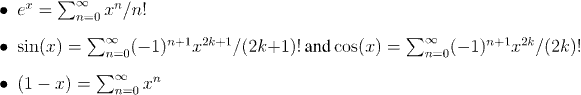Other functions can be expressed as power series too: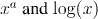are two important examples. In order to verify that these function are equal to power series we will need to know in advance what power series we are trying to show the function is equal to. (Just as, in fact, we needed to know in advance what power series ex was equal to, although we just pulled the answer out of the hat.)

### Mathematical Implications

If a function can be expressed as a power series then the values of that function are completely determined throughout its interval of convergence by the values of its derivatives at a single point. In other words, the behavior of the function at just one point is enough to determine how it will continue to behave, even at points which are far away. Clearly functions of this kind are particularly well-behaved.

Although it is beyond the scope of this course, it is possible to identify which functions are expressible as power series, and the answer comes from complex analysis. The functions which can be expressed as power series are the analytic functions. Essentially, those are the functions which are differentiable (in the sense of complex derivatives) at every point inside an open set.

### Not all Functions are Taylor Series

However, not all functions are equal to their Taylor series. This hardly needs saying; after all, a function which is not differentiable doesn't even have a Taylor series. And a function may have a certain number of derivatives, but no higher derivative, at x0.

Even for those functions which have derivatives of all orders, there's a more subtle possibility. It might be that the Taylor series exists, and even converges for all values of x, but not to the value of f(x)!

In Homework 4 we show that this is possible. In fact, we exhibit a non-zero function whose Taylor series is zero.

### Taylor's Theorem

Remarkably enough, there is a theorem which allows us to make estimates of how well the partial sums of the Taylor series approximate the original function. (Of course, if we are dealing with a function whose Taylor Series does not converge to the original function, then the approximations will never be very good!)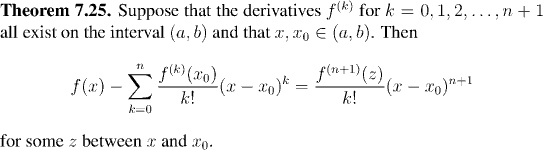Remark:
In principle, this can tell when a function is equal to its Taylor series. What needs to be done is to show that the difference between the function and the partial sums of its Taylor series converges to zero. The difference term, as expressed in Taylor's theorem can sometimes be estimated to show this. The following corollary gives one example: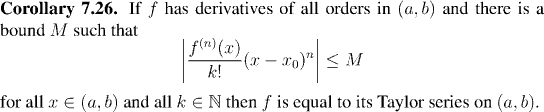Remark:
For the proof, just note that we have shown that the remainder term in Taylor's theorem is dominated by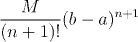which converges to zero.

Unfortunately the condition imposed on f to ensure convergence is very strong. The functions sin(x) and cos(x) satisfy this condition but (apart from polynomials) no other common function can be shown to be equal to its Taylor series from this test. Nevertheless, using other methods, all of the common functions of analysis can be expressed in terms of appropriate Taylor series. The next two examples deal with two important cases: